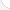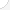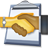Engineering and natural sciences managers Description: Engineering and natural science managers plan and direct research, development, and production in large and small companies and research labs. They hire engineers, chemists, and biologists. They manage and review the work in a business or lab and help determine salaries. They also decide what workers and equipment are needed to do certain jobs.Source: Bureau of Labor Statistics, U.S. Department of Labor, Occupational Outlook Handbook, 2008-09 Edition at http://www.bls.gov/OCO/Complete Job Profile: http://www.bls.gov/oco/ocos009.htmSalary: \$50,001 or more per yearComments:I am a Land Development Engineer/Manager and specialize in private commercial development and civil engineering. I use most of these topics on a daily basis; the more exotic ones are used but considerably less often. Hope this helps you. - bluestreak@cfl.rr.com (Roger Walters)

There are 62 math topics Engineering and natural sciences managers need to know.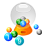Basic Math / Algebra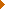FractionsDecimalsRatio and ProportionPercentCustomary MeasurementMetric MeasurementMeasurement ConversionBasic ProbabilityBasic StatisticsStatistical GraphingPowers and RootsOther Number BasesNegative NumbersScientific NotationBasic Problem Solving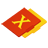First-Year AlgebraUsing FormulasLinear EquationsLinear InequalitiesOperations with PolynomialsFactoring PolynomialsRational ExpressionsCoordinate Graphing 2DLinear SystemsRadicalsQuadratic EquationsAlgebraic Representation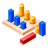GeometryBasic TerminologyAngle MeasurementCongruent TrianglesTriangle InequalitiesParallel LinesQuadrilateralsSimilarityGeometric MeanPythagorean TheoremRight Triangle TrigonometryCirclesConstructionsAreaVolumeTransformationsMake/Use 3D Drawings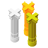Second-Year Algebra / TrigonometryFunctionsVariationPolynomial EquationsLogarithmsCoordinate Graphing 3DTrigonometric/Circular FunctionsGraphs of Trigonometric FunctionsTrigonometric IdentitiesTrigonometric Equations/InversesVectors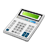Other TopicsCalculus and Higher MathBasic Calculator UseScientific Calculator UseComputer UseComputer ProgrammingGroup Problem SolvingMental MathInductive/Deductive ReasoningMath CommunicationsMathematical Modeling